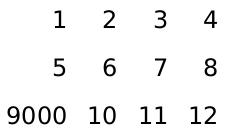### 3Pict Combiners

 procedure(vl-append [d] pict ...) → pict? d : real? = 0.0 pict : pict-convertible? (vc-append [d] pict ...) → pict? d : real? = 0.0 pict : pict-convertible? (vr-append [d] pict ...) → pict? d : real? = 0.0 pict : pict-convertible? (ht-append [d] pict ...) → pict? d : real? = 0.0 pict : pict-convertible? (htl-append [d] pict ...) → pict? d : real? = 0.0 pict : pict-convertible? (hc-append [d] pict ...) → pict? d : real? = 0.0 pict : pict-convertible? (hbl-append [d] pict ...) → pict? d : real? = 0.0 pict : pict-convertible? (hb-append [d] pict ...) → pict? d : real? = 0.0 pict : pict-convertible?
Creates a new pict as a column (for v...-append) or row (for h...-append) of other picts. The optional d argument specifies amount of space to insert between each pair of pictures in making the column or row.

Different procedures align pictures in the orthogonal direction in different ways. For example, vl-append left-aligns all of the pictures.

The descent of the result corresponds to baseline that is lowest in the result among all of the picts’ descent-specified baselines; similarly, the ascent of the result corresponds to the highest ascent-specified baseline. If at least one pict is supplied, then the last element (as reported by pict-last) for the result is (or (pict-last pict) pict) for the using last supplied pict.

Examples:
 (define combiners (list vl-append vc-append vr-append ht-append htl-append hc-append hbl-append hb-append))
 (define names (list "vl-append" "vc-append" "vr-append" "ht-append" "htl-append" "hc-append" "hbl-append" "hb-append"))
 (define pict-a (colorize (filled-rectangle 60 30) "tomato"))
 (define pict-b (colorize (disk 45) "cornflower blue"))
 (define picts (for/list ([combiner combiners] [name names]) (list (text name null 15) (combiner pict-a pict-b))))
> (take picts 4)

'((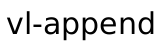) (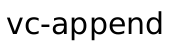) (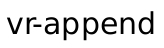) (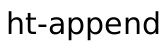))

> (drop picts 4)

'((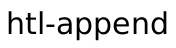) (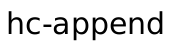) (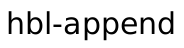) (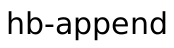))

 procedure(lt-superimpose pict ...) → pict? pict : pict-convertible? (ltl-superimpose pict ...) → pict? pict : pict-convertible? (lc-superimpose pict ...) → pict? pict : pict-convertible? (lbl-superimpose pict ...) → pict? pict : pict-convertible? (lb-superimpose pict ...) → pict? pict : pict-convertible? (ct-superimpose pict ...) → pict? pict : pict-convertible? (ctl-superimpose pict ...) → pict? pict : pict-convertible? (cc-superimpose pict ...) → pict? pict : pict-convertible? (cbl-superimpose pict ...) → pict? pict : pict-convertible? (cb-superimpose pict ...) → pict? pict : pict-convertible? (rt-superimpose pict ...) → pict? pict : pict-convertible? (rtl-superimpose pict ...) → pict? pict : pict-convertible? (rc-superimpose pict ...) → pict? pict : pict-convertible? (rbl-superimpose pict ...) → pict? pict : pict-convertible? (rb-superimpose pict ...) → pict? pict : pict-convertible?
Creates a new picture by superimposing a set of pictures. The name prefixes are alignment indicators: horizontal alignment then vertical alignment.

The descent of the result corresponds to baseline that is lowest in the result among all of the picts’ descent-specified baselines; similarly, the ascent of the result corresponds to the highest ascent-specified baseline. The last element (as reported by pict-last) for the result is the lowest, right-most among the last-element picts of the pict arguments, as determined by comparing the last-element bottom-right corners.

Examples:
 (define combiners (list lt-superimpose  ltl-superimpose lc-superimpose lbl-superimpose lb-superimpose  ct-superimpose ctl-superimpose cc-superimpose  cbl-superimpose cb-superimpose  rt-superimpose  rtl-superimpose rc-superimpose  rbl-superimpose rb-superimpose))
 (define names (list "lt-superimpose"  "ltl-superimpose" "lc-superimpose" "lbl-superimpose" "lb-superimpose"  "ct-superimpose" "ctl-superimpose" "cc-superimpose"  "cbl-superimpose" "cb-superimpose"  "rt-superimpose"  "rtl-superimpose" "rc-superimpose"  "rbl-superimpose" "rb-superimpose"))
 (define pict-a (colorize (filled-rectangle 60 30) "tomato"))
 (define pict-b (colorize (disk 45) "cornflower blue"))
 (define picts (for/list ([combiner combiners] [name names]) (list (text name null 15) (combiner pict-a pict-b))))
> (take picts 3)

'((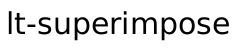) (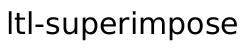) (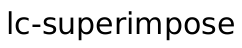))

> (take (drop picts 3) 3)

'((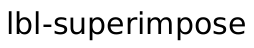) (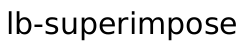) (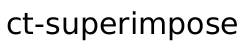))

> (take (drop picts 6) 3)

'((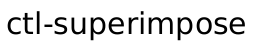) (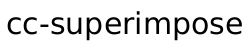) (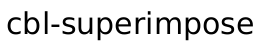))

> (take (drop picts 9) 3)

'((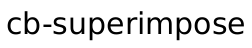) (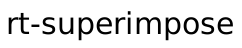) (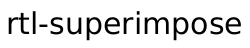))

> (take (drop picts 12) 3)

'((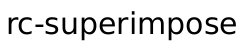) (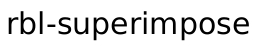) (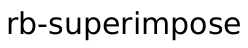))

 procedure(pin-over base dx dy pict) → pict? base : pict-convertible? dx : real? dy : real? pict : pict-convertible? (pin-over base find-pict find pict) → pict? base : pict-convertible? find-pict : pict-path? find : (pict-convertible? pict-path? . -> . (values real? real?)) pict : pict-convertible?
Creates a pict with the same bounding box, ascent, and descent as base, but with pict placed on top. The dx and dy arguments specify how far right and down the second pict’s corner is from the first pict’s corner. Alternately, the find-pict and find arguments find a point in base for find-pict; the find procedure should be something like lt-find.

Examples:
 > (pin-over (colorize (filled-rectangle 70 40) "chocolate") 10 10 (colorize (filled-rectangle 30 30) "orange"))> (define top (colorize (filled-rectangle 70 40) "royalblue"))
 > (pin-over (vc-append top (colorize (filled-rectangle 70 40) "firebrick")) top cb-find (colorize (disk 20) "white"))procedure(pin-under base dx dy pict) → pict? base : pict-convertible? dx : real? dy : real? pict : pict-convertible? (pin-under base find-pict find pict) → pict? base : pict-convertible? find-pict : pict-convertible? find : (pict-convertible? pict-path? . -> . (values real? real?)) pict : pict-convertible?
Like pin-over, but pict is drawn before base in the resulting combination.

Examples:
 > (define txt (colorize (text "P I C T S" null 25) "chocolate"))
 > (define rect (colorize (filled-rectangle (pict-width txt) (* 0.3 (pict-height txt))) "lemonchiffon"))
 > (pin-under txt 0 (- (/ (pict-height txt) 2) (/ (pict-height rect) 2)) rect)procedure

 (table ncols picts col-aligns row-aligns col-seps row-seps) → pict?
ncols : exact-positive-integer?
picts : (non-empty-listof pict-convertible?)
col-aligns :
 (or/c (list*of (->* () #:rest (listof pict-convertible?) pict-convertible?)) (listof (->* () #:rest (listof pict-convertible?) pict-convertible?)))
row-aligns :
 (or/c (list*of (->* () #:rest (listof pict-convertible?) pict-convertible?)) (listof (->* () #:rest (listof pict-convertible?) pict-convertible?)))
col-seps : (or/c (list*of real?) (listof real?))
row-seps : (or/c (list*of real?) (listof real?))
Creates a table given a list of picts. The picts list is a concatenation of the table’s rows (which means that a Racket list call can be formatted to reflect the shape of the output table).

The col-aligns, row-aligns, col-seps, and row-seps arguments are “lists” specifying the row and columns alignments separation between rows and columns. For c columns and r rows, the first two should have c and r superimpose procedures, and the last two should have c-1 and r-1 numbers, respectively. The lists can be “improper” (i.e., ending in a number instead of an empty list), in which case the non-pair cdr is used as the value for all remaining list items that were expected. The col-aligns and row-aligns procedures are used to superimpose all of the cells in a column or row; this superimposition determines the total width or height of the column or row, and also determines the horizontal or vertical placement of each cell in the column or row.

Examples:
 > (table 4 (map (λ (x) (text (format "~a" x))) (list 1 2 3 4 5 6 7 8 9000 10 11 12)) cc-superimpose cc-superimpose 10 10)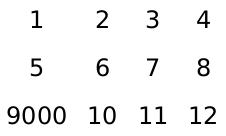> (table 4 (map (λ (x) (text (format "~a" x))) (list 1 2 3 4 5 6 7 8 9000 10 11 12)) rc-superimpose cc-superimpose 10 10)